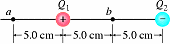# Voltage potential due to 2 charges

## Homework Statement

Find the potential at points a and b in the diagram for charges Q1 = +1.60 nC and Q2 = -1.60 nC.

I know b is 0V = kQ/r

## The Attempt at a Solution

I tried this using the equation and got

8.99e9 * [( +1.60e-6 / .05) + ( -1.60e-6 / .15 ) ] = 191700 V but this isn't right any suggestions??

Kurdt
Staff Emeritus
Gold Member

## Homework Statement

Find the potential at points a and b in the diagram for charges Q1 = +1.60 nC and Q2 = -1.60 nC.

I know b is 0V = kQ/r

## The Attempt at a Solution

I tried this using the equation and got

8.99e9 * [( +1.60e-6 / .05) + ( -1.60e-6 / .15 ) ] = 191700 V but this isn't right any suggestions??

If its nancoulombs as the question suggests then you've just made a numerical error in the solution. Nano = x10-9

Wow how'd I miss that thanks!